# How to Add Spaces between Numbers in Excel?

When you collect data from another source and use that in an Excel sheet, it may not look good. The space provided between numbers in an Excel cell is very less and it will not be very clear. There is a need for increasing the space between the numbers in the cell to represent the numbers neatly. You can use Formulas in Excel to add more space between numbers. In this tutorial, we will take an example to show how you can use a simple formula to add space between numbers in cells and make them more presentable.

## Adding Spaces between Numbers in Excel

Let's suppose we have an Excel sheet with the following data. We will use a Formula to insert spaces at specified places to format the numbers in a certain way: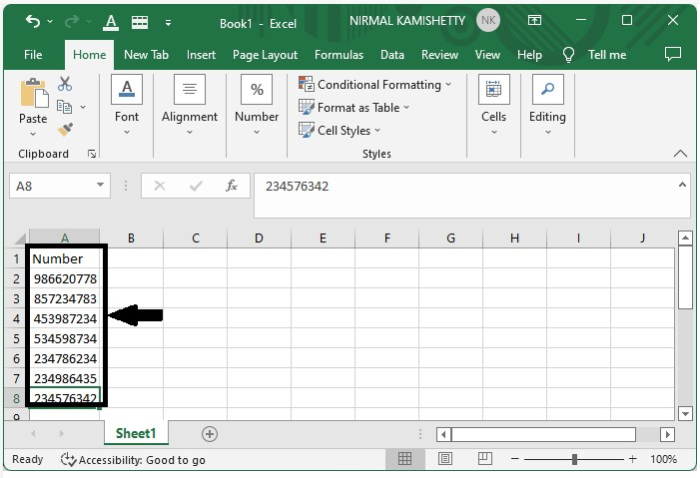Put the cursor on the Formula textbox on the Quick Access Toolbar and enter the formula to insert spaces.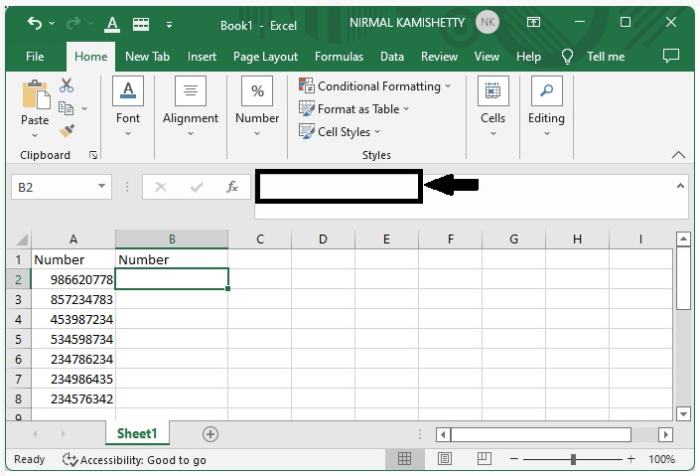The formula for inserting spaces between the numbers is: =TEXT(A2,"### #### ####"). Here we are formatting the number in the cell "A2". Enter the formula in the textbox as shown in the image below.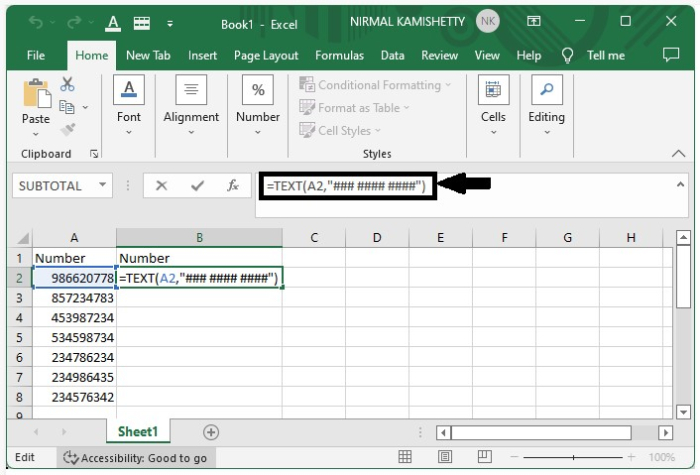After inserting the given Formula, hit the "Enter" button to get the first output.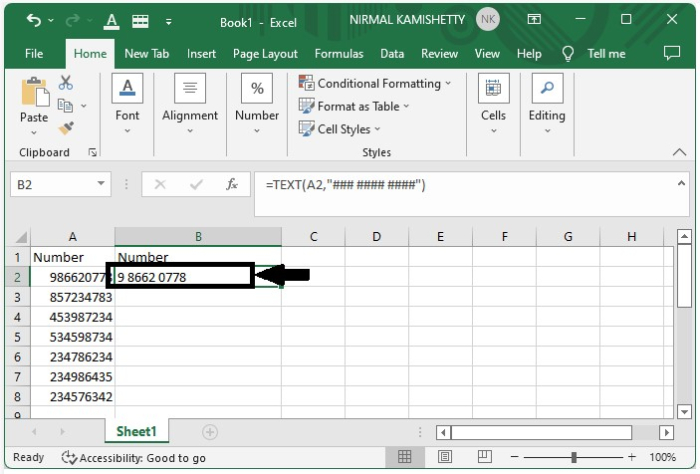We have successfully created the solution for the first cell. Now all that is needed to be done is to select the cell "A2" and drag it down from its right corner to apply the same formatting style in all the other cells. Our final result will look like the one shown below: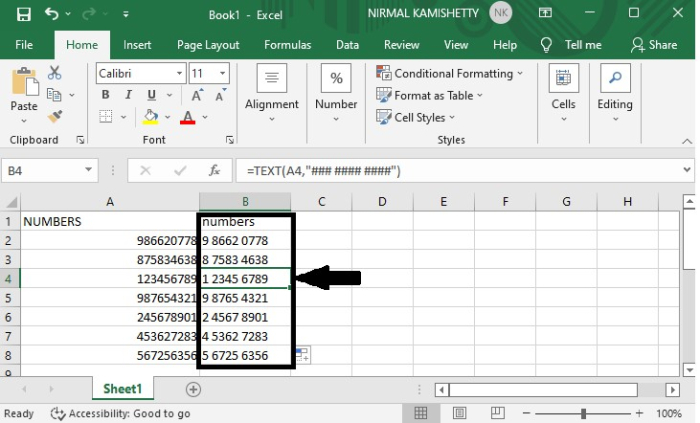The arrangement of numbers will depend on the number of "#" symbols you use in the formula. You can change the grouping just by changing the sequence of "#" symbols in the formula.

## Conclusion

In this tutorial, we demonstrated how you can use Formulas in Excel to insert spaces between numbers and format them the way you like.